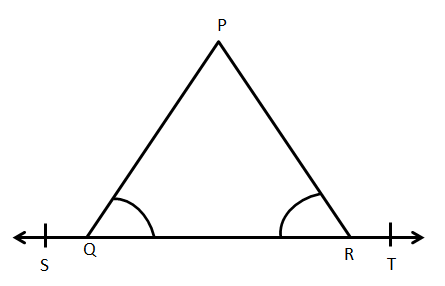# If $\angle PQR = \angle PRQ$Then prove that $\angle PQS = \angle PRT$Verified
177.9k+ views
Hint: Here, we will use the property of linear pairs to find the value of the given angles. Since, the given angles are equal, we will equate their value. After equating and cancelling out the like terms, we will find that $\angle PQS = \angle PRT$, hence, proving the given condition.

We know that by the property of linear pair,
$\angle PQR + \angle PQS = 180^\circ$
Subtracting $\angle PQS$ on both the sides, we get
$\Rightarrow \angle PQR = 180^\circ - \angle PQS$…………………………………………….$\left( 1 \right)$
Similarly, by the property of linear pair,
$\angle PRQ + \angle PRT = 180^\circ$
Subtracting $\angle PRT$ on both the sides, we get
$\Rightarrow \angle PRQ = 180^\circ - \angle PRT$……………………………………………….$\left( 2 \right)$
But, according to the question, it is given that $\angle PQR = \angle PRQ$
Hence, equating $\left( 1 \right)$ and $\left( 2 \right)$, we get,
$180^\circ - \angle PQS = 180^\circ - \angle PRT$
Cancelling out $180^\circ$from both sides, we get
$\Rightarrow - \angle PQS = - \angle PRT$
Again, cancelling out the negative sign from both sides, we get
$\Rightarrow \angle PQS = \angle PRT$
Hence, proved.
Therefore, if $\angle PQR = \angle PRQ$ then by linear pair, $\angle PQS = \angle PRT$

Note: Linear pair is defined as the pair of adjacent angles which are formed when two lines intersect each other. The sum of angles in a linear pair is always supplementary i.e. the angles add up to $180^\circ$. Also, from the word ‘pair’ we can see, that linear pair consists of the sum of two adjacent angles only. In case, we find that three angles are in a linear pair and we know that their sum is $180^\circ$, then also, we cannot state that these angles are $180^\circ$. In such a case, we add those three angles and write that; since, these three angles lie on a straight line, hence, their sum is $180^\circ$. This would be a more valid and accurate reason. Hence, for these types of questions, whenever the internal angles are given equal, we can make their external angles equal by using a linear pair.